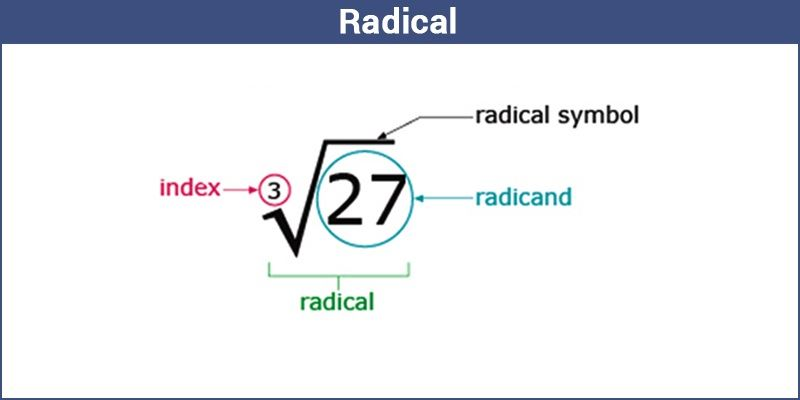A Radical is an expression that involves a root, usually a square root or cube root.

The three different components of radical are;

• Degree
• Symbol

There are three major components of a radical namely:

### Degree

It indicates how many times a radicand is multiplied by itself. If the number is missing on the root symbol, it is assumed as 2(square root).

$$\begin{array}{l}Degree \rightarrow \sqrt{a+b}\end{array}$$

The symbol √ means “root of”. The length of the horizontal bar actually matters.

$$\begin{array}{l}Radical Symbol \rightarrow \sqrt{a+b}\end{array}$$

Note – The horizontal bar in the symbol specifies the extent of the expression has to be operated.

$$\begin{array}{l}\sqrt{x-y}\end{array}$$

In the above expression, the bar is stretched along the whole expression “x – y”. This reveals that you subtract x from y and then find the square root of it.

If it has only covered the ‘x’ like this:

$$\begin{array}{l}\sqrt{x}-y\end{array}$$

It shows that you need to find the square root of x and then subtract y from the result.

This is the one of which you are finding the root. In the expression, √(3x), 3x is the radicand.

A radical equation is the one that has at least one variable expression within a radical, most often the square root.

The radical can be any root, maybe square root, cube root. Generally, you solve equations by isolating the variable by undoing what has been done to it.

For example , given x + 2 = 5. You can solve it by undoing the addition of 2. That is, by applying the opposite.

x + 2 = 5

x  = 5 – 2

x = 3

When you have a variable in a square root, you undo the root by just performing the opposite. For instance, given x = -4 within the root, you can square on both sides and get the resultant as:

(√x)2 = (4)2

x = 16

When it is required to multiply radicals, you need to multiply the numbers outside(o) the radical and then multiply the value inside(i) the radicals.

$$\begin{array}{l}a_{1}\sqrt{I_{1}}.a_{2}\sqrt{I_{2}} = a_{1}a_{2}\sqrt{I_{1}I_{2}}\end{array}$$

A radical equation is the one that has at least one variable expression within a radical, most often the square root.

The radical can be any root, maybe a square root, cube root. Generally, you solve equations by isolating the variable by undoing what has been done to it.